# Difference: SupplyChainMP (13 vs. 14)

#### Revision 142009-10-06 - MichaelOSullivan

Line: 1 to 1

 META TOPICPARENT name="SupplyChains"
<-- Ready to Review -->
Changed:
<
<
%BEGINLATEXPREAMBLE% \usepackage{amsmath} \usepackage{amssymb}
>
>
%BEGINLATEXPREAMBLE% \usepackage{amsmath} \usepackage{amssymb}
%ENDLATEXPREAMBLE%

# Supply Chain Mathematical Programmes

Line: 21 to 19

## Introduction

Changed:
<
<
Throughout a supply chain there are many organisations who are trying to make the best decision for their business. These decisions can be formulated as mathematical programmes. We will introduce a supply chain model that is a simplification of that presented in the Supply Chains introduction. We will formulate the mathematical programmes faced by the organisations at each stage of this supply chain. This simplification can be easily extended to supply chain models with more stages (e.g., see Supply Chains) by inserting more Retailer's Problems into the simplfied model.
>
>
Throughout a supply chain there are many organisations who are trying to make the best decision for their business. These decisions can be formulated as mathematical programmes. We will introduce a supply chain model that is a simplification of that presented in the Supply Chains introduction. We will formulate the mathematical programmes faced by the organisations at each stage of this supply chain. This simplification can be easily extended to supply chain models with more stages (e.g., see Supply Chains) by inserting more Retailer's Problems into the simplified model.
The supply chain model considered here has three stages (shown in Figure 1):
1. The manufacturers;
Changed:
<
<
1. The retailers;
2. The demand markets.
Products are created by the manufacturers, sold to the retailers where they are stored, distributed, etc and then sold again in the demand markets to consumers.
>
>
1. The retailers;
2. The demand markets. Products are created by the manufacturers, sold to the retailers where they are stored, distributed, etc and then sold again in the demand markets to consumers.
Figure 1 A 3-stage supply chain model (adapted from Supply Chain Network Economics)
Changed:
<
<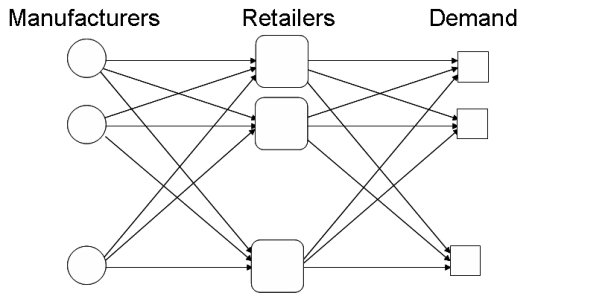>
>The rest of this topic deals with the mathematical programmes faced by the manufacturers, retailers and consumers (in the demand markets).
Line: 39 to 36

## The Manufacturer's Problem

Changed:
<
<
Each manufacturer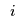(from the set of manufacturers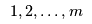must determine an optimal output quantity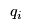which is split into flows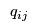to send to each retailer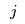(from the set of retailers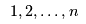). Of course all output is sent to retailers so>
>
Each manufacturer(from the set of manufacturersmust determine an optimal output quantitywhich is split into flowsto send to each retailer(from the set of retailers). Of course all output is sent to retailers soChanged:
<
<
The production cost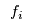for manufacturermay be influenced not only by their output, but also by the outputs from other manufacturers, e.g., due the competition for shared resources. Thus,is a function of the vector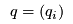which may also be expressed as the matrix of flows from manufacturers to retailers, i.e.,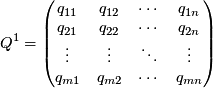so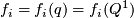Finally, there is a transaction cost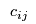associated with sending flowfrom manufacturerto retailer, i.e.,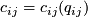Each manufacturersets a unit price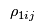for selling their output to retailer. Now manufacturerwant to maximise their profit, i.e., total income - production cost - transaction cost, over the set of nonegative flows: %BEGINLATEX{label="eq:manufacturer"}% \begin{equation*} \begin{array}{l@{ }l} \displaystyle \max \sum_{j=1}^n \rho_{1ij} & \displaystyle q_{ij} - f_i(Q^1) - \sum_{j=1}^n c_{ij}(q_{ij}) \\text{subject to} \& q_{ij} \geq 0, j = 1, 2, \ldots, n \end{array} \end{equation*}

>
>
The production costfor manufacturermay be influenced not only by their output, but also by the outputs from other manufacturers, e.g., due the competition for shared resources. Thus,is a function of the vectorwhich may also be expressed as the matrix of flows from manufacturers to retailers, i.e.,soFinally, there is a transaction costassociated with sending flowfrom manufacturerto retailer, i.e.,Each manufacturersets a unit pricefor selling their output to retailer. Now manufacturerwant to maximise their profit, i.e., total income - production cost - transaction cost, over the set of non-negative flows: %BEGINLATEX{label="eq:manufacturer"}% \begin{equation*} \begin{array}{l@{ }l} \displaystyle \max \sum_{j=1}^n \rho_{1ij} & \displaystyle q_{ij} - f_i(Q^1) - \sum_{j=1}^n c_{ij}(q_{ij}) \ \text{subject to} \ & q_{ij} \geq 0, j = 1, 2, \ldots, n \end{array} \end{equation*}

%ENDLATEX%
Line: 67 to 52
Each retailermust determine their optimal flowto receive from manufacturerand also their optimal flow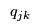to sell to consumers in demand market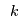(in the set of demand markets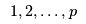).
Changed:
<
<
There is an associated handling cost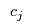that may depend on the flow to other retailers, e.g., competition for output from the manufacturers: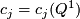>
>
There is an associated handling costthat may depend on the flow to other retailers, e.g., competition for output from the manufacturers:Each retailersets a unit price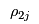for sending flow to a demand market. Note that this price does not depend on the market (as consumers will simply move to the demand markets with the lowest price).

Now the retailer must choose flows to maximise their total profit (total income - handling cost - purchase cost):

Changed:
<
<
%BEGINLATEX{label="eq:retailer"}% \begin{equation*} \begin{array}{l@{ }l@{ }l} \displaystyle \max \rho_{2j} \sum_{k=1}^p & q_{jk} & \displaystyle - \ c_j(Q^1) - \sum_{i=1}^m \rho_{1ij} q_{ij} \\text{subject to} \\displaystyle \hfill \sum_{k=1}^p & q_{jk} & \displaystyle \leq \sum_{i=1}^m q_{ij} \& q_{ij} &\geq 0, i = 1, 2, \ldots, m \& q_{jk} &\geq 0, k = 1, 2, \ldots, p \end{array} \end{equation*}
>
>
%BEGINLATEX{label="eq:retailer"}% \begin{equation*} \begin{array}{l@{ }l@{ }l} \displaystyle \max \rho_{2j} \sum_{k=1}^p & q_{jk} & \displaystyle - \ c_j(Q^1) - \sum_{i=1}^m \rho_{1ij} q_{ij} \ \text{subject to} \ \displaystyle \hfill \sum_{k=1}^p & q_{jk} & \displaystyle \leq \sum_{i=1}^m q_{ij} \ & q_{ij} &\geq 0, i = 1, 2, \ldots, m \ & q_{jk} &\geq 0, k = 1, 2, \ldots, p \end{array} \end{equation*}
%ENDLATEX%

## The Consumers' Problem

Changed:
<
<
The consumers within a demand marketmust determine how much of the incoming flow to purchase. They take the pricefrom each retailer and there is an associated unit transaction cost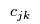for purchasing flow from retailer. This cost may depend on the behaviour of consumers in other demand markets, e.g., . competition for flows from retailers, i.e.,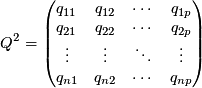and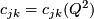>
>
The consumers within a demand marketmust determine how much of the incoming flow to purchase. They take the pricefrom each retailer and there is an associated unit transaction costfor purchasing flow from retailer. This cost may depend on the behaviour of consumers in other demand markets, e.g., . competition for flows from retailers, i.e.,andThe combination of the different prices and unit transaction costs gives rise to an "effective" price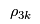for a consumer to purchase a unit of flow in demand market.
Changed:
<
<
The demand for flow in demand marketis determined by the demand curve, a function of the effective price, i.e.,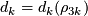>
>
The demand for flow in demand marketis determined by the demand curve, a function of the effective price, i.e.,The consumers within demand marketchoose flows to minimise their total cost while meeting demand:
Changed:
<
<
%BEGINLATEX{label="eq:consumer"}% \begin{equation*} \begin{array}{l@{ }l@{ }l} \displaystyle \min \rho_{3k} \sum_{j=1}^n & q_{jk} \\text{subject to} \\displaystyle \hfill \sum_{j=1}^n & q_{jk} & \geq d_k(\rho_{3k}) \& q_{jk} &\geq 0, j = 1, 2, \ldots, n \end{array} \end{equation*}
>
>
%BEGINLATEX{label="eq:consumer"}% \begin{equation*} \begin{array}{l@{ }l@{ }l} \displaystyle \min \rho_{3k} \sum_{j=1}^n & q_{jk} \ \text{subject to} \ \displaystyle \hfill \sum_{j=1}^n & q_{jk} & \geq d_k(\rho_{3k}) \ & q_{jk} &\geq 0, j = 1, 2, \ldots, n \end{array} \end{equation*}
%ENDLATEX%

## Equilibrium and Variational Inequalities

Changed:
<
<
If the prices,and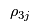are not coordinated then solving the Manufacturer's Problem, the Retailer's Problem and the Consumers' Problem will give inconsistent values forand. That is, the manufacturers will want to ship different ammounts to the retailers than the retailers will want to receive from the maunfacturers and the retailers will want to sell different amounts to the demand markets from the amounts that the consumers at the demand markets want to purchase. However, these prices will adjust until the supply chain reaches equilibrium and the flows from the different mathematical programmes agree.
>
>
If the prices,andare not coordinated then solving the Manufacturer's Problem, the Retailer's Problem and the Consumers' Problem will give inconsistent values forand. That is, the manufacturers will want to ship different amounts to the retailers than the retailers will want to receive from the manufacturers and the retailers will want to sell different amounts to the demand markets from the amounts that the consumers at the demand markets want to purchase. However, these prices will adjust until the supply chain reaches equilibrium and the flows from the different mathematical programmes agree.
One way to find this equilibrium point is to solve a variational inequality. Theorem 1 gives the necessary variational inequalities:

Theorem 1: Variational Inequality The equilibrium state governing the supply chain model according to the optimality conditions of the supply chain mathematical programmes (?? REFLATEX{eq:manufacturer} not defined in eqn list ??), (?? REFLATEX{eq:retailer} not defined in eqn list ??) and (?? REFLATEX{eq:consumer} not defined in eqn list ??) is equivalent to the solution of the variational inequality problem given by: determine the equilibrium vectors of product shipments, shadow prices, and demand market prices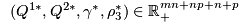satisfying

Changed:
<
<
%BEGINLATEX% \begin{equation*} \begin{split} & \sum_{i=1}^m \sum_{j=1}^n \left[ \frac{\partial f_i(Q^{1*})}{\partial q_{ij}} + \frac{\partial c_{ij}(q_{ij}^*)}{\partial q_{ij}} + \frac{\partial c_j(Q^{1*})}{\partial q_{ij}} - \gamma_j^* \right] \times \left[ q_{ij} - q_{ij}^* \right] \&+ \sum_{j=1}^n \sum_{k=1}^p \left[ c_{jk}(Q^{2*}) + \gamma_j^* - \rho_{3k}^* \right] \times \left[ q_{jk} - q_{jk}^* \right] \&+ \sum_{j=1}^n \left[ \sum_{i=1}^m q_{ij}^* - \sum_{k=1}^p q_{jk}^* \right] \times \left[ \gamma_j - \gamma_j^* \right] \&+ \sum_{k=1}^p \left[ \sum_{j=1}^n q_{jk}^* - d_k(\rho_3^*) \right] \times \left[ \rho_{3k} - \rho_{3k}^* \right] \geq 0, \forall (Q^{1}, Q^{2}, \gamma, \rho_{3}) \in \mathbb{R}_+^{mn+np+n+p} \end{split} \end{equation*}
>
>
%BEGINLATEX% \begin{equation*} \begin{split} & \sum_{i=1}^m \sum_{j=1}^n \left[ \frac{\partial f_i(Q^{1*})}{\partial q_{ij}} + \frac{\partial c_{ij}(q_{ij}^*)}{\partial q_{ij}} + \frac{\partial c_j(Q^{1*})}{\partial q_{ij}} - \gamma_j^* \right] \times \left[ q_{ij} - q_{ij}^* \right] \ &+ \sum_{j=1}^n \sum_{k=1}^p \left[ c_{jk}(Q^{2*}) + \gamma_j^* - \rho_{3k}^* \right] \times \left[ q_{jk} - q_{jk}^* \right] \ &+ \sum_{j=1}^n \left[ \sum_{i=1}^m q_{ij}^* - \sum_{k=1}^p q_{jk}^* \right] \times \left[ \gamma_j - \gamma_j^* \right] \ &+ \sum_{k=1}^p \left[ \sum_{j=1}^n q_{jk}^* - d_k(\rho_3^*) \right] \times \left[ \rho_{3k} - \rho_{3k}^* \right] \geq 0, \forall (Q^{1}, Q^{2}, \gamma, \rho_{3}) \in \mathbb{R}_+^{mn+np+n+p} \end{split} \end{equation*}
%ENDLATEX%

Once the equilibrium state has been found the manufacturers' prices and the retailers' prices can be calculated:

Line: 168 to 123

 META FILEATTACHMENT attachment="latexc4fcd1f7e80fa349b24cfaaf68ec5d28.png" attr="h" comment="" date="1207048331" name="latexc4fcd1f7e80fa349b24cfaaf68ec5d28.png" stream="GLOB(0x8e875a0)" tmpFilename="latexc4fcd1f7e80fa349b24cfaaf68ec5d28.png" user="MichaelOSullivan" version="1" attachment="latex109b7ce4252e22e2189597f1798107c1.png" attr="h" comment="" date="1207049196" name="latex109b7ce4252e22e2189597f1798107c1.png" stream="GLOB(0xa89a574)" tmpFilename="latex109b7ce4252e22e2189597f1798107c1.png" user="MichaelOSullivan" version="1" attachment="latex813a7e6cd57411a1b867f121059a868c.png" attr="h" comment="" date="1207049196" name="latex813a7e6cd57411a1b867f121059a868c.png" stream="GLOB(0xa89a580)" tmpFilename="latex813a7e6cd57411a1b867f121059a868c.png" user="MichaelOSullivan" version="1"
Deleted:
<
<
 META FILEATTACHMENT attachment="latexb5912e945e9329efa3deca443764042e.png" attr="h" comment="" date="1207049824" name="latexb5912e945e9329efa3deca443764042e.png" stream="GLOB(0xaaba7ac)" tmpFilename="latexb5912e945e9329efa3deca443764042e.png" user="MichaelOSullivan" version="1" attachment="latex26d615107ca4c212aad3419fc2885034.png" attr="h" comment="" date="1207049824" name="latex26d615107ca4c212aad3419fc2885034.png" stream="GLOB(0xaaba818)" tmpFilename="latex26d615107ca4c212aad3419fc2885034.png" user="MichaelOSullivan" version="1" attachment="latex533779377b97fd3c43de6837161d5078.png" attr="h" comment="" date="1207049824" name="latex533779377b97fd3c43de6837161d5078.png" stream="GLOB(0xaaba758)" tmpFilename="latex533779377b97fd3c43de6837161d5078.png" user="MichaelOSullivan" version="1" attachment="latexa9c92bcfdc81177b4d2b139f7fa8b7f4.png" attr="h" comment="" date="1207050261" name="latexa9c92bcfdc81177b4d2b139f7fa8b7f4.png" stream="GLOB(0x9ad7050)" tmpFilename="latexa9c92bcfdc81177b4d2b139f7fa8b7f4.png" user="MichaelOSullivan" version="1"

 META FILEATTACHMENT attachment="latexdbde51c32699928974582b4a0d99f300.png" attr="h" comment="" date="1207050541" name="latexdbde51c32699928974582b4a0d99f300.png" stream="GLOB(0xa739abc)" tmpFilename="latexdbde51c32699928974582b4a0d99f300.png" user="MichaelOSullivan" version="1" attachment="latex59f03a8f6b43496526f96f273a850e5b.png" attr="h" comment="" date="1207050542" name="latex59f03a8f6b43496526f96f273a850e5b.png" stream="GLOB(0xa739b28)" tmpFilename="latex59f03a8f6b43496526f96f273a850e5b.png" user="MichaelOSullivan" version="1" attachment="latex1e01a2903b6d8b4f47b4e06511bb3e5f.png" attr="h" comment="" date="1207050542" name="latex1e01a2903b6d8b4f47b4e06511bb3e5f.png" stream="GLOB(0xa739a68)" tmpFilename="latex1e01a2903b6d8b4f47b4e06511bb3e5f.png" user="MichaelOSullivan" version="1"

Copyright © 2008-2023 by the contributing authors. All material on this collaboration platform is the property of the contributing authors.
Ideas, requests, problems regarding TWiki? Send feedback